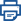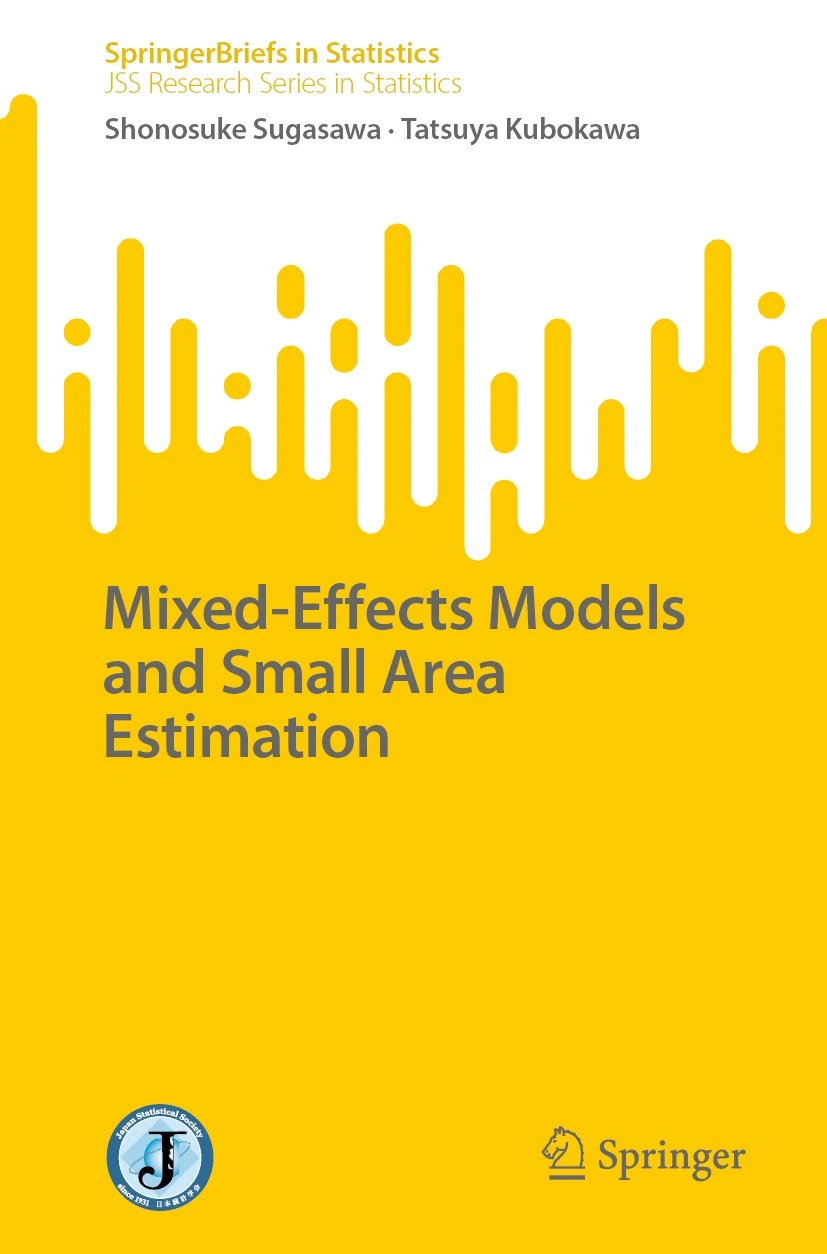Print this pageTitle

# SpringerBriefs in Statistics (JSS Research Series in Statistics)Mixed-Effects Models and Small Area Estimation

Size

121 pages, softcover

Language

English

Released

February 04, 2023

ISBN

978-981-19-9485-2

Springer Singapore

Book Info

See Book Availability at Library

Japanese Page

view japanese page

This book provides a self-contained introduction to the technical aspects of mixed-effects models and small area estimation. It places special emphasis on introducing classical theory and reviewing the latest methodologies. It begins by introducing fundamental issues of mixed-effects models, such as parameter estimation, random effects prediction, variable selection, and asymptotic theory. It then goes on to describe the standard mixed-effects models used in small area estimation, specifically the Fay-Herriot model and the nested error regression model, and presents both the frequentist and Bayesian approaches. Methods for measuring the uncertainty of these small area estimators, including mean squared errors and confidence interval calculations, are discussed. The book covers a range of advanced techniques that use mixed-effects models, including both frequentist and Bayesian approaches; All these aspects make the book valuable for researchers and graduate students in the various fields that require data analysis skills and a grasp of mathematical statistics.

The term “small area” refers to geographically limited regions, such as counties, municipalities, or states, as well as specific demographic groups defined by age, gender, or race. When estimating characteristics of such small groups, direct estimation based solely on the data from these groups can be unreliable. This creates the problem of small area estimation, seeking reliable estimates for characteristics within such groups. Due to an increasing demand for trustworthy small area estimates across public and private sectors, this kind of estimation has been actively researched from both theoretical and practical perspectives. A linear mixed-effects model, incorporating relevant supplementary information as covariates, is employed to enhance the accuracy of small area estimates. This results in empirical best linear unbiased predictors (EBLUP), offering reliable estimates for small area characteristics.

The book’s first part details the derivation of mean squared errors, prediction intervals, and second-order asymptotic unbiased estimation for EBLUP in general linear mixed models. It provides concrete examples by applying these to the Fay-Herriot and nested error regression models, which are commonly used in small area estimation. Furthermore, techniques for hypothesis testing and variable selection in linear mixed models are introduced.

The book's latter part shifts focus to advanced small area estimation techniques based on mixed-effects models and is supplemented with practical examples. Chapter 6 delves into advanced theories for fundamental small area models, focusing on addressing practical problems. Techniques covered include adjusted likelihood methods for estimating random effects variance, observed best prediction for random effects, and robust prediction and fitting of small area models. Chapter 7 introduces strategies for dealing with non-normal response variables in small area estimation, incorporating generalized linear mixed models, models with data transformation, and models involving non-normal distributions. Finally, Chapter 8 reviews extensions of fundamental small area models, encompassing topics like flexible modeling of random effects, measurement error models, nonparametric and semiparametric models, and models with heteroscedastic variance.

(Written by KUBOKAWA Tatsuya, Professor, Graduate School of Economics / 2023)

1. Introduction
2. General Mixed-Effects Models and BLUP
3. Measuring Uncertainty of Predictors
4. Basic Mixed-Effects Models for Small Area Estimation
5. Hypothesis Tests and Variable Selection
6. Advanced Theory of Basic Small Area Models
7. Small Area Models for Non-normal Response Variables
8. Extensions of Basic Small Area Models

## Related Info

Book Review:
By Desak Made Goldyna Rarasari  (Journal of Agricultural, Biological and Environmental Statistics  16th May 2023)